Latest Banking jobs   »

# Quantitative Aptitude Quiz For FCI Phase I 2022- 14th December

Directions (1-5): – Table given below gives information about Cost price, Selling price, profit percent and discount percentage of four article A, B, C and D. Some data is missing, calculate the data and answer the following questions.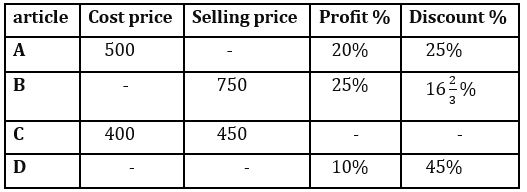Q1. If cost price of article D is 10% more than cost price of article A, find Market price of article D?
(a) Rs. 1000
(b) Rs. 1100
(c) Rs. 900
(d) Rs. 950
(e) Rs. 1050

Q2. Average of market price of article A and cost price of article B is how much percent more than cost price of article C?
(a) 75%
(b) 80%
(c) 60%
(d) 90%
(e) 95%

Q3. If discount percentage is twice of profit percentage for article C, find ratio of cost price of article A to market price of article C?
(a) 2:3
(b) 1:2
(c) 2:5
(d) 5:6
(e) None of these.

Q4. Selling price of article C is what percent of market price of article B?
(a) 100%
(b) 75%
(c) 50%
(d) 25%
(e) 60%

Q5. If for article D difference between profit earned and discount given is Rs. 440, find selling price of the D?
(a) 550
(b) 650
(c) 308
(d) 450
(e) 605

Directions (6-10): – Pie chart given below shows percentage distribution of production of six different grains in a state in a year. Read the data carefully and answer the following questions.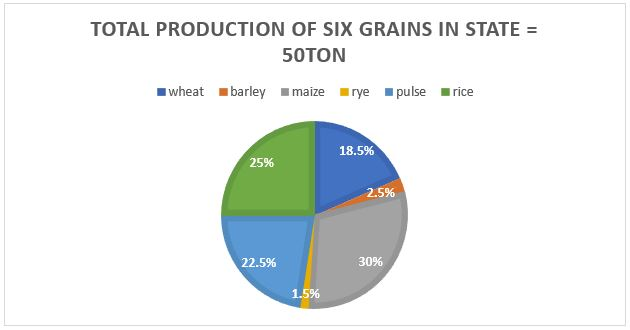Q6. Production of pulse is how much more than production of rye? (in ton)
(a) 10.5
(b) 11
(c) 10.7
(d) 9.5
(e) 8.4

Q7. What is the ratio of production of barley and rice together to that of wheat and maize together?
(a) 11:13
(b) 55:97
(c) 13:97
(d) 22:39
(e) None of these.

Q8. If price of a Kg of barley is Rs.35 and price of a Kg of pulse is Rs. 80, if both are mixed in ratio of their production in given state, find price of per kg of mixture formed?
(a) Rs.75
(b) Rs.72.5
(c) Rs.60
(d) Rs.75.5
(e) Rs. 68

Q9. If production of wheat and maize is increased by 20% and 25% percent respectively in next year but the total production of these six grains remain the same, find total increase in production of wheat and maize together in next year?
(a) 10400 kg
(b) 5600 kg
(c) 6400 kg
(d) 7500 kg
(e) 7200 kg

Q10. What is the central angle formed by production of rice? (in degree)
(a) 45
(b) 120
(c) 90
(d) 60
(e) 75

Directions (11-15): – Pie chart given below shows percentage break up of revenue generated by six different items (LED, refrigerator, cable, laptops, mobile and calculator) of a shop in a month? (assume shop sell only these six items)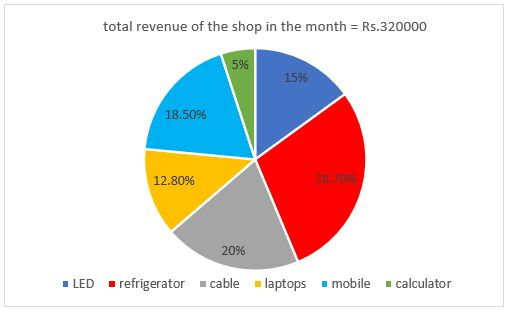Q11. Revenue from refrigerator is how much more than revenue from laptops?
(a) Rs.40960
(b) Rs.64000
(c) Rs.59200
(d) Rs.50880
(e) Rs.48000

Q12. What is the difference between central angle (in degree) formed by revenue from refrigerator, laptops and mobile together and revenue from cable?
(a) 144
(b) 108
(c) 72
(d) 180
(e) 240

Q13. Revenue from mobile is how much percent more than revenue from calculator?
(a) 370%
(b) 225%
(c) 250%
(d) 275%
(e) 270%

Q14. If total 4 LED were sold in that month and selling price of each laptop in that month is Rs.5120, find no. of laptop sold is how much percent more than no. of LED sold in that month?
(a) 50%
(b) 100%
(c) 25%
(d) 75%
(e) 200%

Q15. What is the total revenue (in Rs.) from cable and mobile together?
(a) 223100
(b) 123000
(c) 123200
(d) 148200
(e) None of these.

Solutions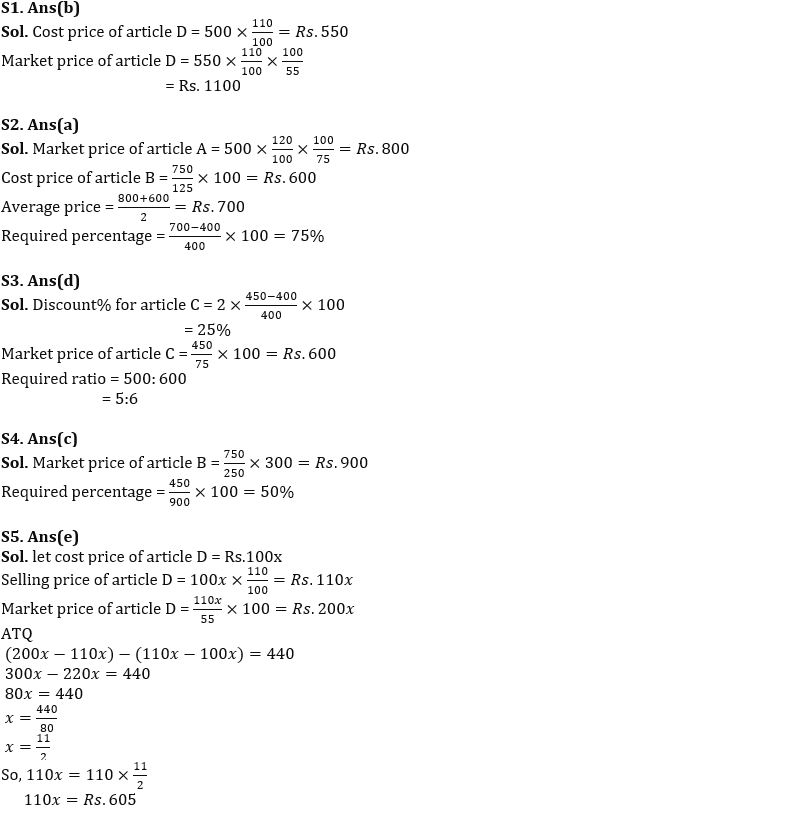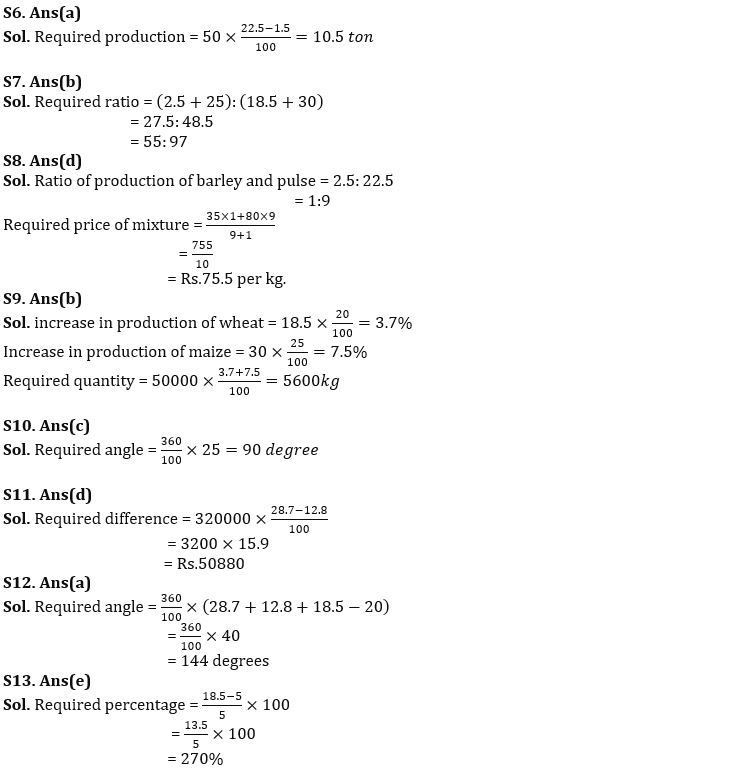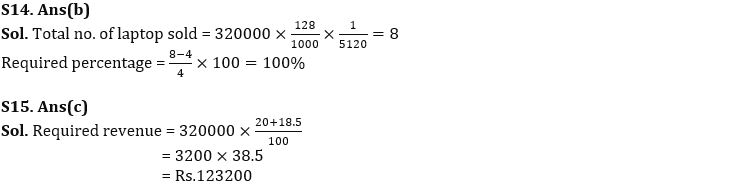#### Congratulations!Union Budget 2023-24: Free PDF